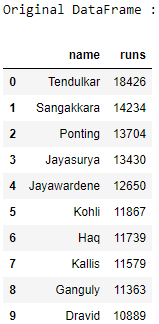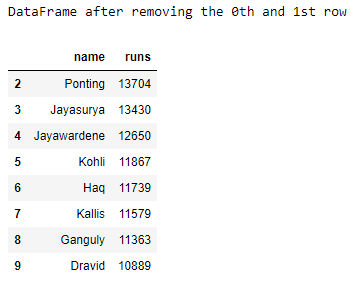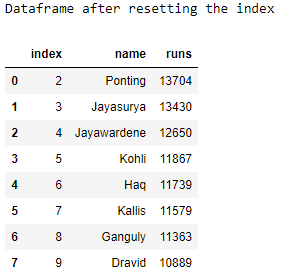# Pandas – How to reset index in a given DataFrame

• Last Updated : 25 Aug, 2021

Let us see how to reset the index of a DataFrame after dropping some of the rows from the DataFrame.
Approach :

1. Import the Pandas module.
2. Create a DataFrame.
3. Drop some rows from the DataFrame using the drop() method.
4. Reset the index of the DataFrame using the reset_index() method.
5. Display the DataFrame after each step.

## Python3

 `# importing the modules``import` `pandas as pd``import` `numpy as np` `# creating a DataFrame``ODI_runs ``=` `{``'name'``: [``'Tendulkar'``, ``'Sangakkara'``, ``'Ponting'``,``                      ``'Jayasurya'``, ``'Jayawardene'``, ``'Kohli'``,``                      ``'Haq'``, ``'Kallis'``, ``'Ganguly'``, ``'Dravid'``],``            ``'runs'``: [``18426``, ``14234``, ``13704``, ``13430``, ``12650``,``                     ``11867``, ``11739``, ``11579``, ``11363``, ``10889``]}``df ``=` `pd.DataFrame(ODI_runs)` `# displaying the original DataFrame``print``(``"Original DataFrame :"``)``print``(df)` `# dropping the 0th and the 1st index``df ``=` `df.drop([``0``, ``1``])` `# displaying the altered DataFrame``print``(``"DataFrame after removing the 0th and 1st row"``)``print``(df)` `# resetting the DataFrame index``df ``=` `df.reset_index()` `# displaying the DataFrame with new index``print``(``"Dataframe after resetting the index"``)``print``(df)`

Output :My Personal Notes arrow_drop_up